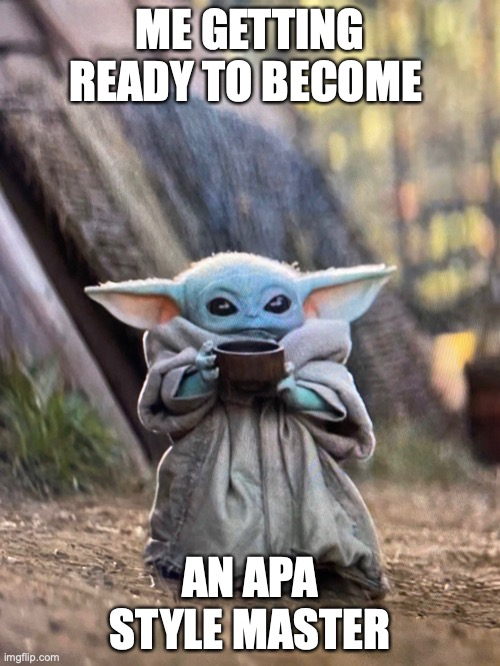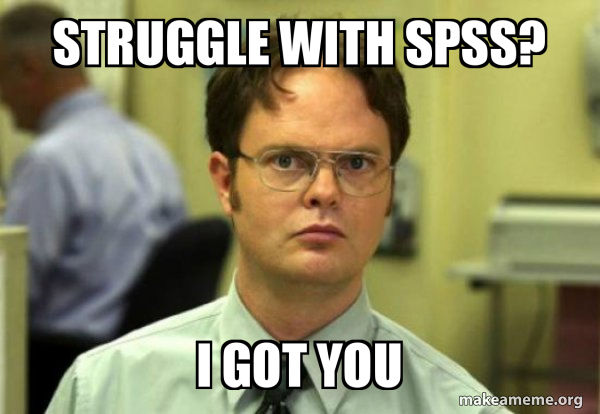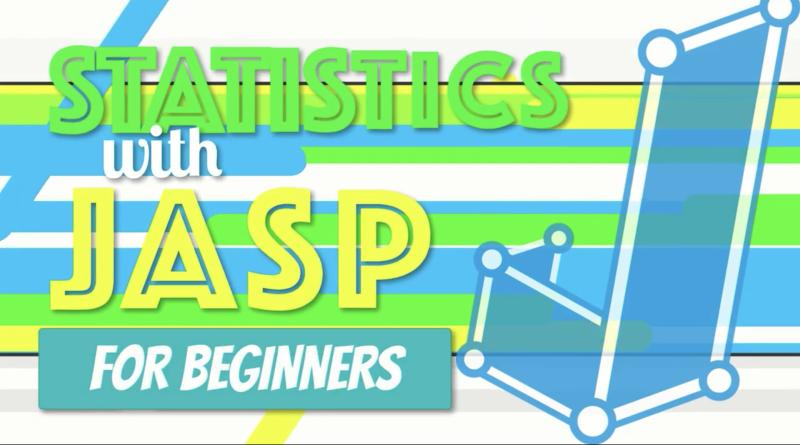# Guide/Tutorial## Student Guide to SPSS and APA Style Writing (v.12.0)

This guide (created by Gary Lewandowski; Twitter: @LewandowskiPhD) is a free resource that can substitute for the APA Manual and SPSS guides. The Student Guide leads students through data analysis in SPSS with step-by-step instructions and screenshots for setting up the analyses and interpreting the output. For each analysis, students also see an example SPSS …## APA Style (7th Edition): Writing the Discussion (Key Phrases to Use)

Anyone who has ever taught writing of APA Style Research Reports, knows that students struggle with writing the Discussion section. The APA now provides a handout that helps students by providing key phrases for each subsection. These should help students better match their content to the appropriate section. You can find the handout here.## APA Style (7th Edition): Academic Writer Tutorial: Basics of Seventh Edition APA Style

This is an online guide from the APA that introduces APA Style writing and formatting. This would be a great resource to introduce students to APA, especially for an online course. From the site “By the end of this tutorial, you should be able to understand and implement the following basic elements of APA Style” …## Video: One-Way ANOVA (SPSS for Beginners)

Video (11:19) from Research by Design described as follows, “Using the same example from the Wizard of Oz involving Munchkins and wicked witches in various regions that we used learning ANOVA by hand, we are going to learn about conducting a one-way ANOVA using SPSS. We will create the dataset in SPSS, conduct a one-way …## Video: Chi-Square (SPSS for Beginners)

Video (7:37) from Research by Design described as follows, “We are now going to learn how to calculate a one-way chi-square in SPSS. This is the House of Pigs Chi Square, using the dataset BuildingPermits.sav. A house inspector randomly samples building permits pulled by 70 little pigs who were building houses, to determine whether a …## Video: Independent-Samples t-test (SPSS for Beginners)

Video (8:11) from Research by Design and Dr. Todd Daniel, described as follows, “Another way of measuring the difference between two samples is to compare two unrelated groups or participants or samples. In this design, you measure two groups one time; in contrast, the previous paired test measured the same sample two times. With independent …## Video: One Sample and Paired-Samples t-test (SPSS for Beginners)

Video (6:02) from Research by Design and Dr. Todd Daniel, described as follows, “When we calculate the mean of a sample, we can then use a one-sample t test to compare that sample mean to another mean, such as a mean from a population, a normative group, or another known value (mean). The “one sample” …## Video: Correlation (SPSS for Beginners)

Video (9:52) from Research by Design and Dr. Todd Daniel, described as follows, “Using the dataset we created in the first video, we now explore the relationship between our two scale-level variables: height and weight. We will use Pearson’s correlation to measure this relationship. We will also learn about a point biserial correlation with gender.” …## Video: Frequencies and Descriptive Statistics (SPSS for Beginners)

Video (14:01) from Research by Design and Dr. Todd Daniel, described as follows, “Having learned how to create a variable, you are ready to begin entering data. Dr. Daniel begins with descriptive statistics (numbers that describe our data) and shows you how to do some basic data cleaning and data exploration with the Frequencies command …## Video: Introduction to SPSS & Creating Variables (SPSS for Beginners)

Video (10:15) from Research by Design and Dr. Todd Daniel, described as follows, “Are you ready to learn how to use SPSS for your introductory statistics class? You have come to the right place. This is the first in a series of eight videos that will introduce you to using SPSS for introductory statistics. This …## Video: How to Do Chi-Square in JASP

Video (10:27) from Research by Design in which, “We learn how to calculate a One-Way Chi-Square goodness of fit test in JASP using the setting for Multinomial Test. For the null hypothesis, we assume that the observed values in one group (the Pigs) do not differ from the values in a comparison population (the Bears) …## Video: How to Do Simple Linear Regression in JAS

Video (23:18) from Research by Design in which, “Exploring our data about burnout and job satisfaction, we predict an outcome with a single variable using simple linear regression in JASP. I explain how regression works, then open an SPSS dataset in JASP. We explore the assumptions of homoscedasticity and linearity with a scatterplot and normality …## Video: How to Do a Pearson Correlation in JASP

Video (18:47) from Research by Design in which, “Using a dataset about burnout and job satisfaction, we learn how to conduct a simple correlation, how to interpret it, and how to write it up in APA style. We begin by exploring the theoretical relationship among the variables to understand how correlation works. After we walk …## Video: How to Do an One-Way ANOVA in JASP

Video (14:02) from Research by Design in which, “We are off to see the wizard with an example of a one-way (factorial) ANOVA involving wicked witches and munchkins. We open a .CSV dataset in JASP, check the assumptions of homogeneity of variance and normality, conduct the test, interpret the results, and write up the findings …## Video: How to Do an Independent Samples t Test in JASP

Video (18:29) from Research by Design in which, “Using a dataset about puppy training, we learn how to set up and run an independent samples t test. We import data into JASP, conduct the test, interpret the results and write up the findings. We will learn to check the assumptions of homogeneity of variance using …## Video: How to Do a One Sample and Paired-Samples t Test in JASP

Video (16:57) from Research by Design in which, “The One Sample t-Test is a parametric procedure that tests whether a sample mean is statistically significantly different than a population mean or other known value. We will open a CSV dataset in JASP, check the assumptions of the test, conduct the one sample t-test, interpret the …